Latest Banking jobs   »   Quantitative Aptitude Quiz For RRB PO...

# Quantitative Aptitude Quiz For RRB PO Mains 2021- 4th September

Q1. Shikha and Sameer have equal amount. Shikha invested on C.I. for two years at the rate of 10% p.a. and Sameer invested 66 ⅔% of amount at the rate of R% p.a. on simple interest and remaining amount at the rate of 6.5% p.a. on simple interest. If interest received by both at the end of two years are equal, then find the value of ‘R’?
(a) 10%
(b) 12.5%
(c) 15%
(d) 12%
(e) 8%

Q2. A sold a pen to B at Rs 60 profit. ‘B’ increased its marked price by 50% and then sold it to C at a discount of 25%. Profit earned by ‘B’ is Rs 10 more than A. Find A’s cost price?
(a) 250
(b) 500
(c) 750
(d) 1000
(e) 1250

Q3. There are four light poles in four corners of a park, four at midpoint of each side and one pole in the middle of that square park. Area of park is 19600 cm2. If side of park is 50 times of radius of each light pole, then find the total base area covered by these poles if pole’s base is circular in shape?
(a) 197.12 cm²
(b) 172.48 cm²
(c) 246.4 cm²
(d) 220.76 cm²
(e) 221.76 cm²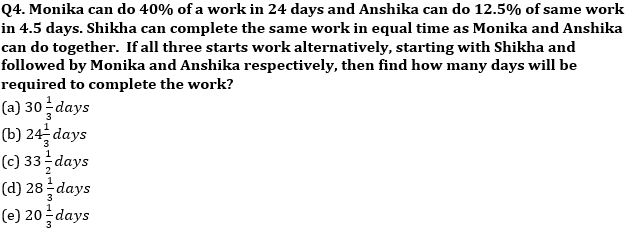Q5. A started a business with Rs 9000 and B joined him with Rs 16000 after 3 months. After a year ‘A’ got 35% of total profit for his managerial work while remaining profit is divided into A and B according to their investment. It after a year ‘A’ got Rs 52800 as his share, the total profit earned by them?
(a) 74,000
(b) 76,000
(c) 80,000
(d) 84,000
(e) 88,000

Directions (6-10): The following questions are accompanied by two statements (I) and (II). You have to determine which statements(s) is/are sufficient/necessary to answer the questions.
(a) Statement (I) alone is sufficient to answer the question but statement (II) alone is not sufficient to answer the questions.
(b) Statement (II) alone is sufficient to answer the question but statement (I) alone is not sufficient to answer the question.
(c) Both the statements taken together are necessary to answer the questions, but neither of the statements alone is sufficient to answer the question.
(d) Either statement (I) or statement (II) by itself is sufficient to answer the question.
(e) Statements (I) and (II) taken together are not sufficient to answer the question.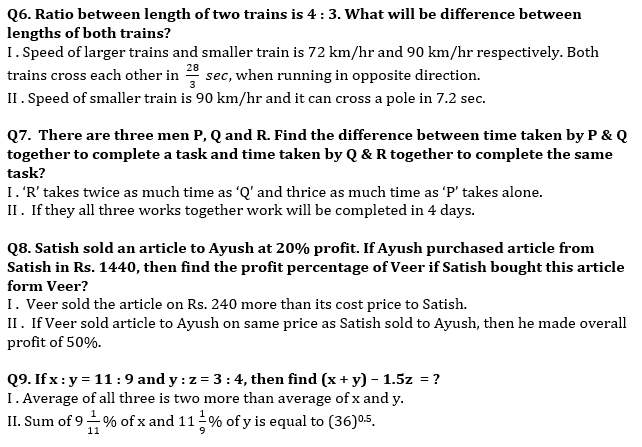Q10. Ratio between length and breadth of rectangle ‘X’ is 7 : 4. Find area of a square ‘Y’?
I . length of rectangle ‘X’ is two times of radius of circle, whose area is 616 cm².
II . Perimeter of rectangle ‘X’ is 20 cm more than perimeter of square ‘Y’.

Solutions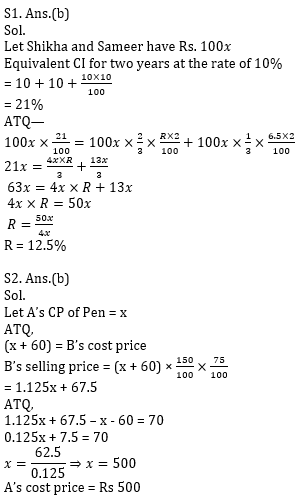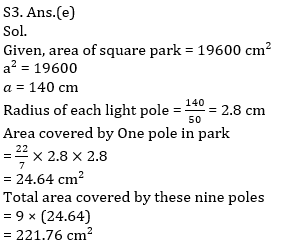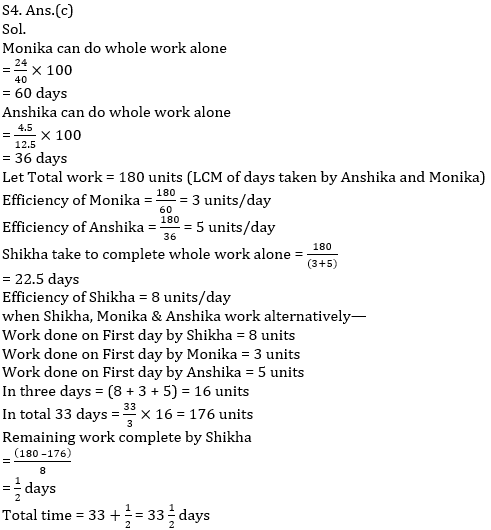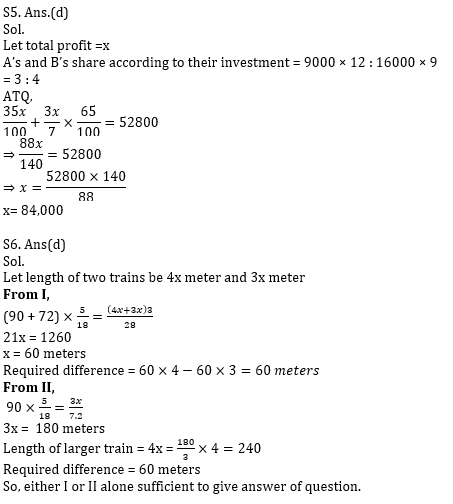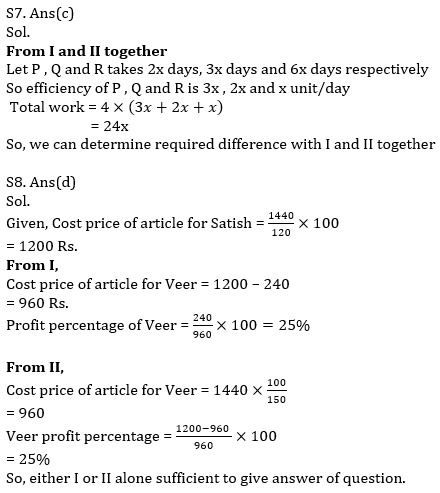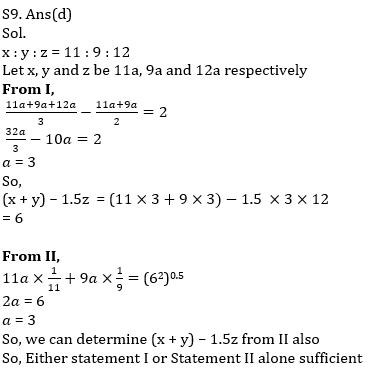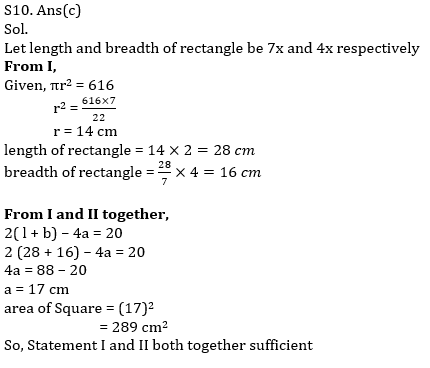Click Here to Register for Bank Exams 2021 Preparation Material#### Congratulations!Download Hindu Review of October 2021: Free PDF